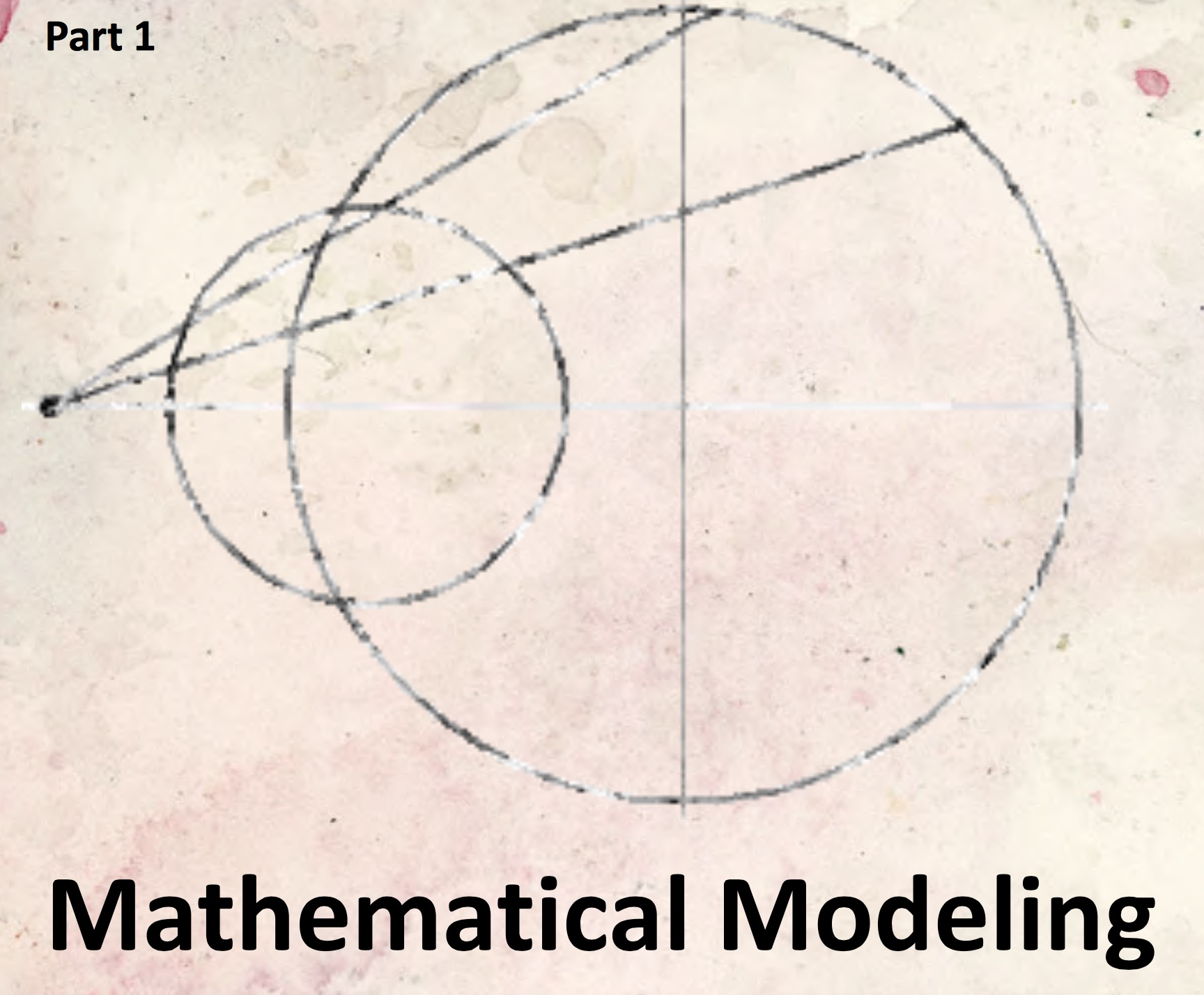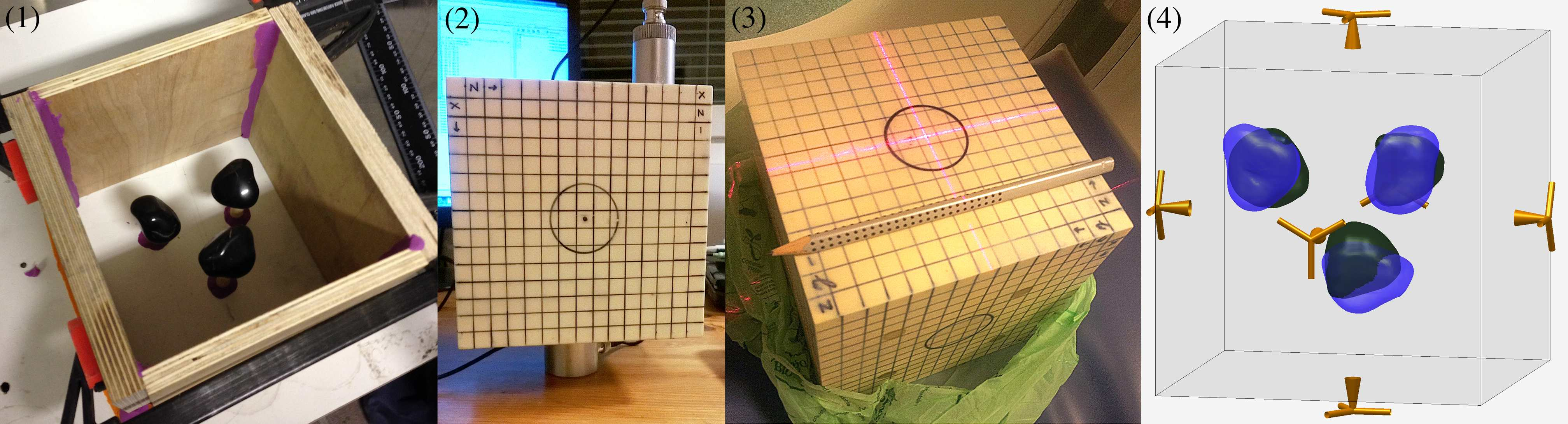## Conformal mappings, numerical analysis and mathematical modeling

Antti Rasila Department of Mathematics and Systems Analysis Aalto University Conformal mappings are a class of functions studied in the classical complex analysis, which are defined by the property that they preserve angles between smooth curves. The basic properties of the complex multiplication along with the chain rule give another […]

## Finnish National Network on Mathematical ModellingThe Finnish National Network on Mathematical Modelling was founded by the following Finnish universities and their departments (in the alphabetical order). http://math.tut.fi/eu-maths-in/ Lappeenranta University of Technology (LUT), School of Engineering Science. Tampere University of Technology (TUT), Department of Mathematics University of Eastern Finland (UEF) Department of Applied Physics (Kuopio) University […]

## Finnish-Chinese eLearning on Mathematical Modeling: modules on PDE’s and ODE’s.Mathematical modeling and simulation form a crucial method for applying mathematics in practice. With the development of computers and software it has grown into a pivotal R&D-method throughout science. The importance of modeling has been internationally recognized and it has been included into the curricula of many universities. […]

## Online education for mathematical modelling in Finland

Seven Finnish universities have joined their efforts to teach mathematical modelling in Finland with the help of the World Wide Web. By bundling the resources and expertise, they managed to offer modelling courses that would otherwise be beyond the capabilities of the individual universities. The collaboration started 2002 as a […]